nichols

Nichols chart of frequency response

Description

example

nichols(sys) creates a Nichols chart of the frequency response of a dynamic system model sys. The plot displays the magnitude (in dB) and phase (in degrees) of the system response as a function of frequency. nichols automatically determines frequencies to plot based on system dynamics. Use ngrid to superimpose Nichols chart grid lines on an existing SISO Nichols chart.

If sys is a multi-input, multi-output (MIMO) model, then nichols produces an array of Nichols charts, each plot showing the frequency response of one I/O pair.

If sys is a model with complex coefficients, then nichols plot shows a contour comprised of both positive and negative frequencies. For models with real coefficients, nichols only shows positive frequencies.

example

nichols(sys1,sys2,...,sysN) plots the Nichols plot of the frequency response of multiple dynamic systems on the same plot. All systems must have the same number of inputs and outputs.

example

nichols(sys1,LineSpec1,...,sysN,LineSpecN) specifies a color, line style, and marker for each system in the plot.

example

nichols(___,w) plots the response for frequencies specified by w.

• If w is a cell array of the form {wmin,wmax}, then nichols plots the chart at frequencies ranging between wmin and wmax.

• If w is a vector of frequencies, then nichols plots the chart at each specified frequency. The vector w can contain both negative and positive frequencies.

You can use w with any of the input-argument combinations in previous syntaxes.

example

[mag,phase,wout] = nichols(sys) returns the magnitude and phase of the response at each frequency in the vector wout. The function automatically determines frequencies in wout based on system dynamics. This syntax does not draw a plot.

example

[mag,phase,wout] = nichols(sys,w) returns the response data at the frequencies specified by w.

• If w is a cell array of the form {wmin,wmax}, then wout contains frequencies ranging between wmin and wmax.

• If w is a vector of frequencies, then wout = w.

Examples

collapse all

Plot the Nichols response with Nichols grid lines for the following system:

$H\left(s\right)=\frac{-4{s}^{4}+48{s}^{3}-18{s}^{2}+250s+600}{{s}^{4}+30{s}^{3}+282{s}^{2}+525s+60}.$

H = tf([-4 48 -18 250 600],[1 30 282 525 60]);
nichols(H)
ngrid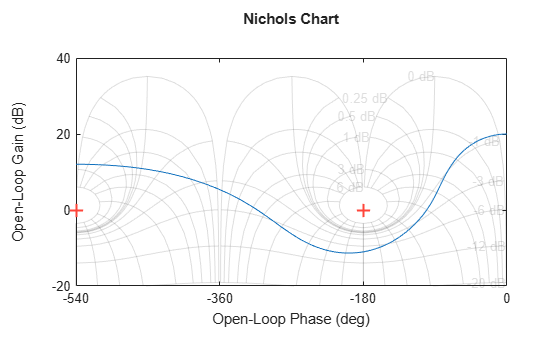The context menu for Nichols charts includes the Tight option under Zoom. You can use this option to clip unbounded branches of the Nichols chart.

Create a Nichols plot over a specified frequency range. Use this approach when you want to focus on the dynamics in a particular range of frequencies.

H = tf([-0.1,-2.4,-181,-1950],[1,3.3,990,2600]);
nichols(H,{1,100})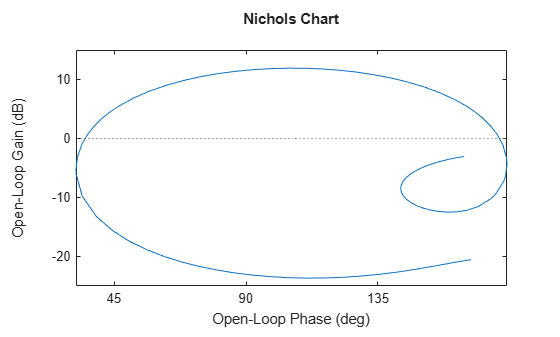The cell array {1,100} specifies the minimum and maximum frequency values in the Nichols plot. When you provide frequency bounds in this way, the function selects intermediate points for frequency response data.

Alternatively, specify a vector of frequency points to use for evaluating and plotting the frequency response.

w = 1:0.5:100;
nichols(H,w,'.-')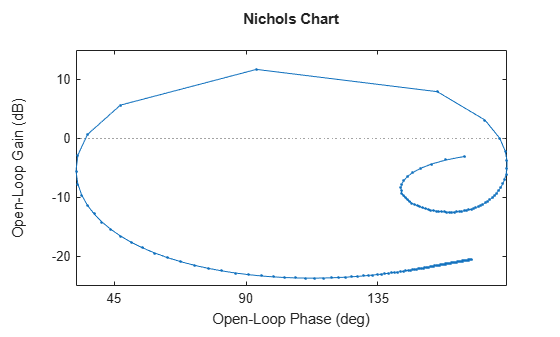nichols plots the frequency response at the specified frequencies only.

Compare the frequency response of a continuous-time system to an equivalent discretized system on the same Nichols plot.

Create continuous-time and discrete-time dynamic systems.

H = tf([1 0.1 7.5],[1 0.12 9 0 0]);
Hd = c2d(H,0.5,'zoh');

Create a Nichols plot that displays both systems.

nichols(H,Hd)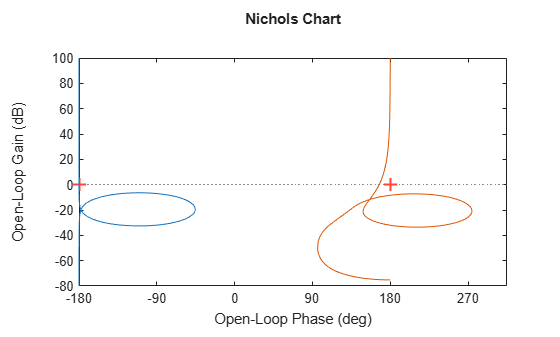Specify the line style, color, or marker for each system in a Nichols plot using the LineSpec input argument.

H = tf([1 0.1 7.5],[1 0.12 9 0 0]);
Hd = c2d(H,0.5,'zoh');
nichols(H,'r',Hd,'b--')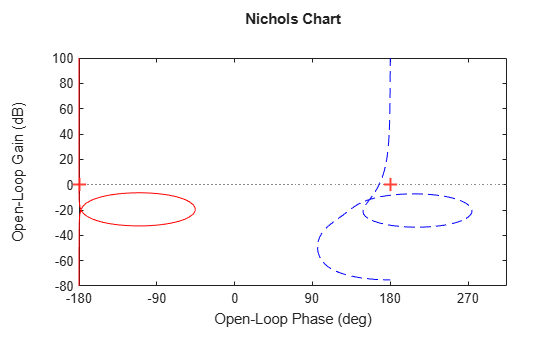The first LineSpec, 'r', specifies a solid red line for the response of H. The second LineSpec, 'b--', specifies a dashed blue line for the response of Hd.

Compute the magnitude and phase of the frequency response of a SISO system.

If you do not specify frequencies, nichols chooses frequencies based on the system dynamics and returns them in the third output argument.

H = tf([1 0.1 7.5],[1 0.12 9 0 0]);
[mag,phase,wout] = nichols(H);

Because H is a SISO model, the first two dimensions of mag and phase are both 1. The third dimension is the number of frequencies in wout.

size(mag)
ans = 1×3

1     1   110

length(wout)
ans = 110

Thus, each entry along the third dimension of mag gives the magnitude of the response at the corresponding frequency in wout.

For this example, create a 2-output, 3-input system.

rng(0,'twister');

For this system, nichols plots the frequency responses of each I/O channel in a separate plot in a single figure.

nichols(H)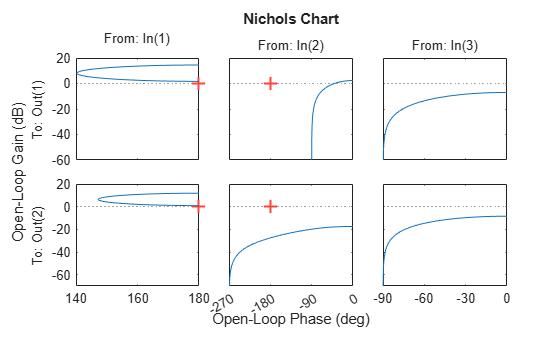Compute the magnitude and phase of these responses at 20 frequencies between 1 and 10 radians.

w = logspace(0,1,20);
[mag,phase] = nichols(H,w);

mag and phase are three-dimensional arrays, in which the first two dimensions correspond to the output and input dimensions of H, and the third dimension is the number of frequencies. For instance, examine the dimensions of mag.

size(mag)
ans = 1×3

2     3    20

Thus, for example, mag(1,3,10) is the magnitude of the response from the third input to the first output, computed at the 10th frequency in w. Similarly, phase(1,3,10) contains the phase of the same response.

Create a Nichols plot of a model with complex coefficients and a model with real coefficients on the same plot.

rng(0)
A = [-3.50,-1.25-0.25i;2,0];
B = [1;0];
C = [-0.75-0.5i,0.625-0.125i];
D = 0.5;
Gc = ss(A,B,C,D);
nichols(Gc,Gr)
legend('Complex-coefficient model','Real-coefficient model','Location','southwest')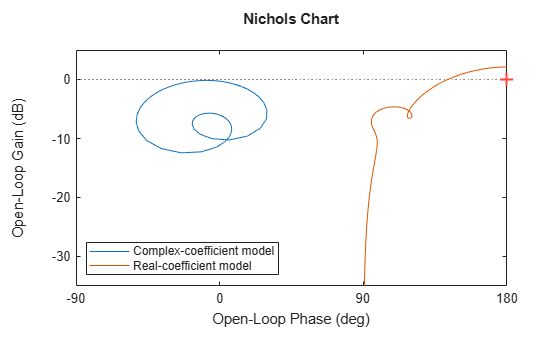For models with complex coefficients, nichols shows a contour comprised of both positive and negative frequencies. For models with real coefficients, the plot shows only positive frequencies, even when complex-coefficient models are present. You can click the curve to further examine which section and values correspond to positive and negative frequencies.

Input Arguments

collapse all

Dynamic system, specified as a SISO or MIMO dynamic system model or array of dynamic system models. Dynamic systems that you can use include:

• Continuous-time or discrete-time numeric LTI models, such as tf, zpk, or ss models.

• Generalized or uncertain LTI models such as genss or uss (Robust Control Toolbox) models. (Using uncertain models requires Robust Control Toolbox™ software.)

• For tunable control design blocks, the function evaluates the model at its current value for both plotting and returning frequency response data.

• For uncertain control design blocks, the function plots the nominal value and random samples of the model. When you use output arguments, the function returns frequency response data for the nominal model only.

• Frequency-response data models such as frd models. For such models, the function plots the response at frequencies defined in the model.

• Identified LTI models, such as idtf (System Identification Toolbox), idss (System Identification Toolbox), or idproc (System Identification Toolbox) models. Using identified models requires System Identification Toolbox™ software.

If sys is an array of models, the function plots the frequency responses of all models in the array on the same axes.

Line style, marker, and color, specified as a string or vector of one, two, or three characters. The characters can appear in any order. You do not need to specify all three characteristics (line style, marker, and color). For example, if you omit the line style and specify the marker, then the plot shows only the marker and no line. For more information about configuring this argument, see the LineSpec input argument of the plot function.

Example: 'r--' specifies a red dashed line

Example: '*b' specifies blue asterisk markers

Example: 'y' specifies a yellow line

Frequencies at which to compute and plot frequency response, specified as the cell array {wmin,wmax} or as a vector of frequency values.

• If w is a cell array of the form {wmin,wmax}, then the function computes the response at frequencies ranging between wmin and wmax.

• If w is a vector of frequencies, then the function computes the response at each specified frequency. For example, use logspace to generate a row vector with logarithmically spaced frequency values. The vector w can contain both positive and negative frequencies.

For models with complex coefficients, if you specify a frequency range of [wmin,wmax] for your plot, then the plot shows a contour comprised of both positive frequencies [wmin,wmax] and negative frequencies [–wmax,–wmin].

Specify frequencies in units of rad/TimeUnit, where TimeUnit is the TimeUnit property of the model.

Output Arguments

collapse all

Magnitude of the system response in absolute units, returned as a 3-D array. The dimensions of this array are (number of system outputs) × (number of system inputs) × (number of frequency points).

To convert the magnitude from absolute units to decibels, use:

magdb = 20*log10(mag)

Phase of the system response in degrees, returned as a 3-D array. The dimensions of this array are (number of outputs)-by-(number of inputs)-by-(number of frequency points).

Frequencies at which the function returns the system response, returned as a column vector. The function chooses the frequency values based on the model dynamics, unless you specify frequencies using the input argument w.

wout also contains negative frequency values for models with complex coefficients.

Frequency values are in radians/TimeUnit, where TimeUnit is the value of the TimeUnit property of sys.